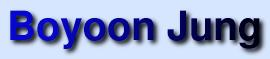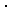Boyoon Jung's Library Manual

bj::ParticleFilter Class Reference [Particle Filter]

A regular particle filter class. More...

#include <particle.h>

Inheritance diagram for bj::ParticleFilter:[legend]
List of all members.

Public Member Functions

ParticleFilter (int num_particles, int dimension, gsl_vector *min=0, gsl_vector *max=0)
A constructor.
~ParticleFilter (void)
A destructor.
void initialize (void)
Initialize a particle filter.
virtual bool update (void)
Update particles.
gsl_vector * spaceMin (void)
Return the lower bound of a state space.
gsl_vector * spaceMax (void)
Return the upper bound of a state space.
void spaceSize (gsl_vector *min, gsl_vector *max)
Return the bounds of a state space.
void setSpaceMin (gsl_vector *min)
Set the lower bound of a state space.
void setSpaceMax (gsl_vector *max)
Set the upper bound of a state space.
void setSpaceSize (gsl_vector *min, gsl_vector *max)
Set the bounds of a state space.
int numParticles (void)
Return the number of particles.
gsl_vector ** particles (void)
Return the set of particles.
double * importances (void)
Return the importance factors.

Protected Member Functions

virtual void propagate (gsl_vector *x, gsl_vector *xnew)=0
Define a dynamics model.
virtual double evaluate (gsl_vector *x)=0
Define a measurement model.
void set_particle_random (gsl_vector *p)
Generate random particles.
int sir (void)
Perform sampling-importance-resampling.

Protected Attributes

gsl_vector ** particle
A set of particles.
double * importance
Importance factors.
int size
The number of particles.
int p_size
The dimension of a particle.
gsl_vector * ss_min
The lower bound of the state space.
gsl_vector * ss_max
The upper bound of the state space.
gsl_rng * rng
Random number generator.
double * cdf
Cumulative density function.
gsl_vector ** p_tmp
Temporary buffer.

Detailed Description

A regular particle filter class.

ParticleFilter class implements a standard particle filter using the static number of particles. A user is supposed to overload the following two abstract functions:

• propagate(x, xnew) := draw a new particle based on a given particle and an action model
• evaluate(x) := evaluate the new particle based on a new measurement

Constructor & Destructor Documentation

 bj::ParticleFilter::ParticleFilter ( int num_particles, int dimension, gsl_vector * min = 0, gsl_vector * max = 0 )

A constructor.

Parameters:
 num_particles The number of particles. dimension The dimension of a particle. min The lower bound of the state space. max The upper bound of the state space.

 bj::ParticleFilter::~ParticleFilter ( void )
 A destructor.

Member Function Documentation

 virtual double bj::ParticleFilter::evaluate ( gsl_vector * x ) [protected, pure virtual]
 Define a measurement model.

 double* bj::ParticleFilter::importances ( void ) [inline]
 Return the importance factors.

 void bj::ParticleFilter::initialize ( void )
 Initialize a particle filter.

 int bj::ParticleFilter::numParticles ( void ) [inline]
 Return the number of particles.

 gsl_vector** bj::ParticleFilter::particles ( void ) [inline]
 Return the set of particles.

 virtual void bj::ParticleFilter::propagate ( gsl_vector * x, gsl_vector * xnew ) [protected, pure virtual]
 Define a dynamics model.

 void bj::ParticleFilter::set_particle_random ( gsl_vector * p ) [inline, protected]
 Generate random particles.

 void bj::ParticleFilter::setSpaceMax ( gsl_vector * max ) [inline]
 Set the upper bound of a state space.

 void bj::ParticleFilter::setSpaceMin ( gsl_vector * min ) [inline]
 Set the lower bound of a state space.

 void bj::ParticleFilter::setSpaceSize ( gsl_vector * min, gsl_vector * max ) [inline]
 Set the bounds of a state space.

 int bj::ParticleFilter::sir ( void ) [protected]
 Perform sampling-importance-resampling.

 gsl_vector* bj::ParticleFilter::spaceMax ( void ) [inline]
 Return the upper bound of a state space.

 gsl_vector* bj::ParticleFilter::spaceMin ( void ) [inline]
 Return the lower bound of a state space.

 void bj::ParticleFilter::spaceSize ( gsl_vector * min, gsl_vector * max ) [inline]
 Return the bounds of a state space.

 virtual bool bj::ParticleFilter::update ( void ) [virtual]

Member Data Documentation

 double* bj::ParticleFilter::cdf [protected]
 Cumulative density function.

 double* bj::ParticleFilter::importance [protected]
 Importance factors.

 int bj::ParticleFilter::p_size [protected]
 The dimension of a particle.

 gsl_vector** bj::ParticleFilter::p_tmp [protected]
 Temporary buffer.

 gsl_vector** bj::ParticleFilter::particle [protected]
 A set of particles.

 gsl_rng* bj::ParticleFilter::rng [protected]
 Random number generator.

 int bj::ParticleFilter::size [protected]
 The number of particles.

 gsl_vector* bj::ParticleFilter::ss_max [protected]
 The upper bound of the state space.

 gsl_vector* bj::ParticleFilter::ss_min [protected]
 The lower bound of the state space.

The documentation for this class was generated from the following file:
ResearchProjectsPublications

ToolsBjLib ManualPlayer/StagePyPlayerMicroStrain IMUGPS/DGPSParticle Filter

PersonalWelcomeCurriculum VitaeResumeContactUSCUSC CS DeptCRESRobotics LabRESL